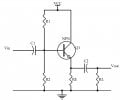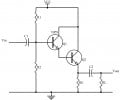# Question regarding darlington pair

#### simpsonss

Joined Jul 8, 2008
173
Hi,

I have a few doubt about the darlington pair characteristic. After reading all the sources, i know that darlington pair has a higher current gain and a low input impedance. Then i start to prove it through experiments.

Figure1 is the common collector by using one transistor. and Figure2 is common collector using a darlington pair. Both has the same resistors value.

Figure1Figure2after calculation i found that, the Figure2 design just increase the input impedance by 4k ohm. And Ic for Figure1 is ~4.7mA while Ic for Figure2 is ~4.37mA. So what i curious about is isnt it Figure2 should have a more higher current compare to Figure1? If not what is the purpose i use the darlington pair since i get almost the same Ic by using the Figure1 design.

This is the question i cant figure out.

Hope someone can help me on this.

thank you.

#### Hi-Z

Joined Jul 31, 2011
158
It's all about input impedance, really - the darlington emitter-follower will give you a much higher input impedance.

The reason is that the input impedance is given by RE in parallel with RL multiplied by the current gain, shunted by R1 in parallel with R2. The feature of the darlington is that current gain is much higher (for idealised transistors it would actually be the square of the current gain of a single normal transistor), so there is potential for much higher input impedance.

Now, if you happen to make R1 and R2 the same for each circuit, and these dominate input impedance, then you won't have gained much by using the darlington. However, because the current gain of the darlington is so much higher, it's possible to increase both R1 and R2 substantially. If you do this (and you should!) then you end up with much higher input impedance, and therefore much less loading of the preceding circuitry.

The emitter current for both circuits is given by the emitter voltage divided by RE. Ic is emitter current minus base current. Base current is lower for the darlington (much higher current gain), so you might perhaps expect the darlington's Ic to be very slightly higher. HOWEVER, the crucial difference is the emitter voltage: since there are 2 Vbe drops associated with the darlington, the emitter voltage will be significantly lower, and therefore the emitter current will be lower.

So, you end up with lower Ic for the darlington (this assumes there is little loading effect on the base resistors for either circuit).

#### studiot

Joined Nov 9, 2007
4,998
To pick out two important points, contrary to simpsonss ideas.

The input impedance is raised, not lowered (and can be very high) by using a darlington.

The bias chain must be different from the chain used for a single transistor to allow for the increased base emitter voltage.

go well

#### t_n_k

Joined Mar 6, 2009
5,455
The question was also raised as to why one would consider using a Darlington pair.

One useful application is in improving the effective β of a low β power transistor. For example, in the case of the series pass control transistor for a linear regulated DC supply.

One would probably be reluctant to use a Darlington in a conventional small signal common emitter amplifier as the OP suggests - even after re-jigging the bias network to obtain similar quiescent conditions . It might appear you get some benefits, but as always there are downsides - such as a much reduced high frequency response.